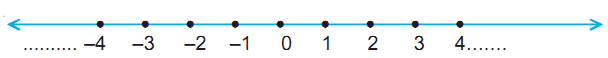# Integers

While dealing with natural numbers and whole numbers, it is not always possible to subtract a number from another. For example, (2 – 3), (3 – 7), and (9 – 20) are all not possible in the system of natural numbers and whole numbers. Thus, it needed another extension of numbers which allow such subtractions.

Thus, we extend whole numbers by such numbers as –1 (called negative 1), –2 (negative 2) and so on.

The integers are ..., – 7, – 6, – 5, – 4, – 3, – 2, – 1, 0, 1, 2, 3, 4, 5, 6, 7, ...

### Number Line

Extend the number line used for representing whole numbers to the left of zero and mark points – 1, – 2, – 3, – 4, ... such that 1 and –1, 2 and –2, 3 and –3 are equidistant from zero and are in opposite directions of zero.For finding the greater (or smaller) of the two integers a and b, follow the following rule:

1. a > b, if a is to the right of b

2. a < b, if a is to the left of b